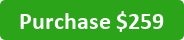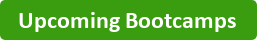Are you new to SQL and starting from scratch but also want to go all the way with your learning? Want to begin as a SQL novice but finish as a proficient user? Then, this Bootcamp allows for you to take and learn all topics covered in all 5 classes from both the Beginner and Intermediate Level Bootcamps.

###Course I: Retrieving Data

• Overview of relational databases and data flow
• Database, Schema, and Tables Overview
• Retrieve Data from a Single Table
• Conversion and explanation of various data types

Course II: Filtering, Sorting, and Aggregating Data

• Utilize conditional operators to filter data
• Sorting data
• Aggregate data using aggregate functions (MIN, MAX, SUM, AVERAGE, COUNT)

Course III: Summarizing Data

• Summarizing data using GROUP BY function

Course IV: Calculating Intermediate Mathematical Operations and Filtering Aggregated Data

• Perform mathematical operations on multiple fields
• Calculate formulas using mathematical operations and aggregate function
• Perform filtering using HAVING vs. WHERE statements
• Use HAVING vs. WHERE statement with mathematical formulas and calculations

Course V: Retrieving Data from Multiple Tables

• Retrieve data from at least 4 tables using Joins